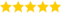# Goto Statement in Python

Last updated on 21st Sep 2020, Artciles, Blog

Krishnan (Sr Technical Project Manager )

He is a TOP-Rated Domain Expert with 6+ Years Of Experience, Also He is a Respective Technical Recruiter for Past 3 Years & Share's this Informative Articles For Freshers(5.0) | 13627 Ratings 720

GOTO is a statement of the early beginnings of programming. It is rarely used in high-level code today.Code that makes use of it is called Spaghetti code by some people. I have almost never seen goto statements in code, so I’ve been curious about them.

### Python

Python does NOT offer GOTO. However, somebody made a GOTO module for April Fools’ Day. See goto in Python if you’re still interested.

### Java

Java has no goto statement. Studies illustrated that goto is (mis)used more often than not simply “because it’s there”. Eliminating goto led to a simplification of the language–there are no rules about the effects of a goto into the middle of a for statement, for example. Studies on approximately 100,000 lines of C code determined that roughly 90 percent of the goto statements were used purely to obtain the effect of breaking out of nested loops. As mentioned above, multi-level break and continue remove most of the need for goto statements.

### GOTO works in C++. Here is a minimal example:

Minimal Example

• int main(){
• int test = 0;
• start:;
• if (test > 10) {
• goto end;
• } else {
• test += 7;
• goto start;
• }
• end:;
• cout << “test: ” << test << endl;
• return 0;
• output;
• test: 14
• }

### Euclidean GCD algorithm

Most of you might know the euclidean algorithm for calculating the greatest common divisor in a version like this one:

• #include <iostream>
• using namespace std;
• int euclidGCD(int a, int b) {
• while (b != 0) {
• int m = a % b;
• a = b;
• b = m;
• }
• return a;
• }
• int main(){
• // Outputs 20
• cout << “GCD of 340 and 32760: ” << euclidGCD(340, 32760) <<endl;
• return 0;
• }

Here is a goto-version that works perfectly fine:

• #include <iostream>
• using namespace std;
• int euclidGCD(int a, int b) {
• if (b > a) goto b_larger;
• while (1) {
• a = a % b;
• if (a == 0) return b;
• b_larger:
• b = b % a;
• if (b == 0) return a;
• }
• }
• int main(){
• cout << “GCD of 340 and 32760: ” << euclidGCD(340, 32760) <<endl;
• return 0;
• }
• #include <iostream>
• using namespace std;
• int myFunction(int i) {
• i += 1;
• inFunctionLabel:;
• i += 1;
• return i;
• }
• int main(){
• int test = 0;
•  goto inFunctionLabel;
•  cout << “test: ” << test << endl;
• return 0;
• }

### Compiler error:

gotoExample.cpp: In function &lsquo;int myFunction(int)&rsquo;:

gotoExample.cpp:7: warning: label &lsquo;inFunctionLabel&rsquo; defined but not used

gotoExample.cpp: In function &lsquo;int main()&rsquo;:

gotoExample.cpp:15: error: label &lsquo;inFunctionLabel&rsquo; used but not defined

So goto is at least bound to its scope.

Are you looking training with Right Jobs?